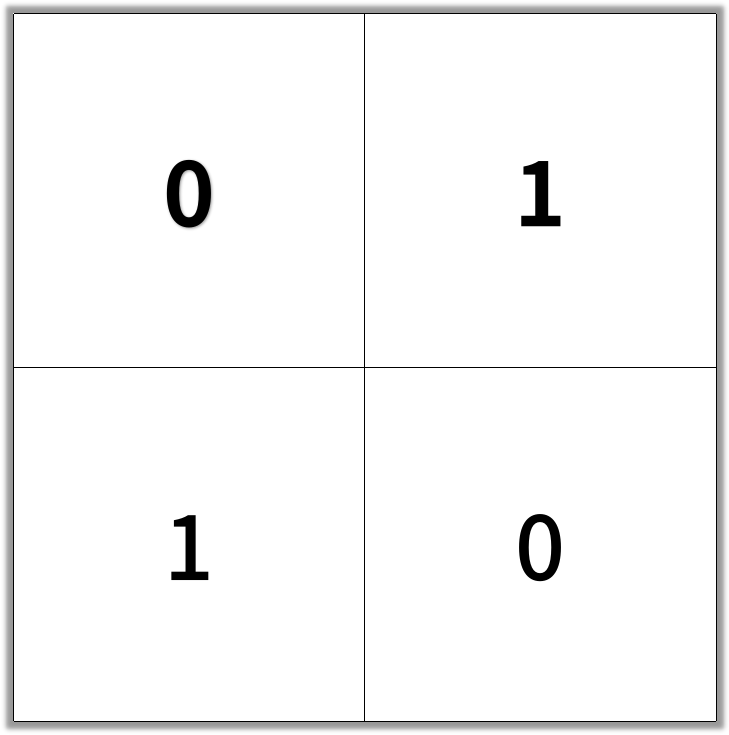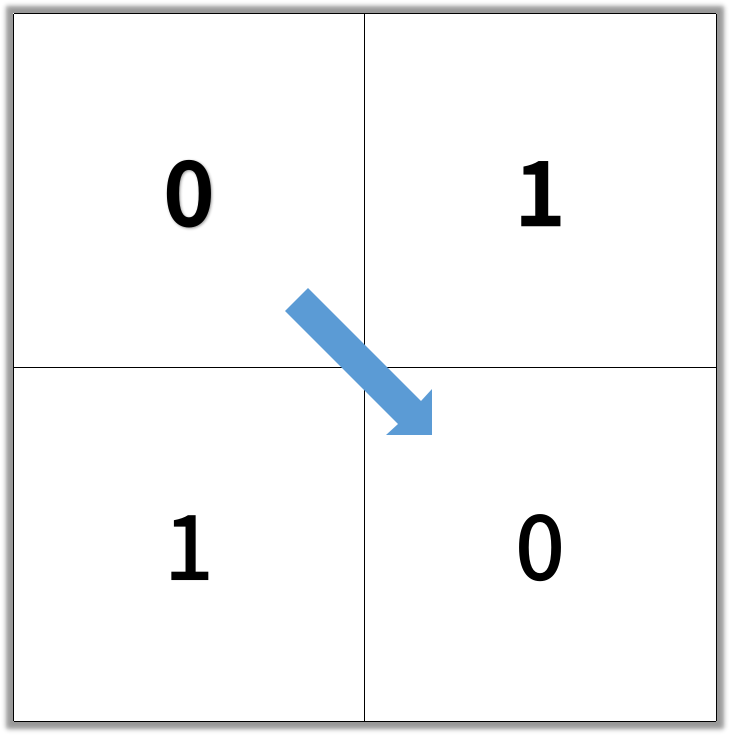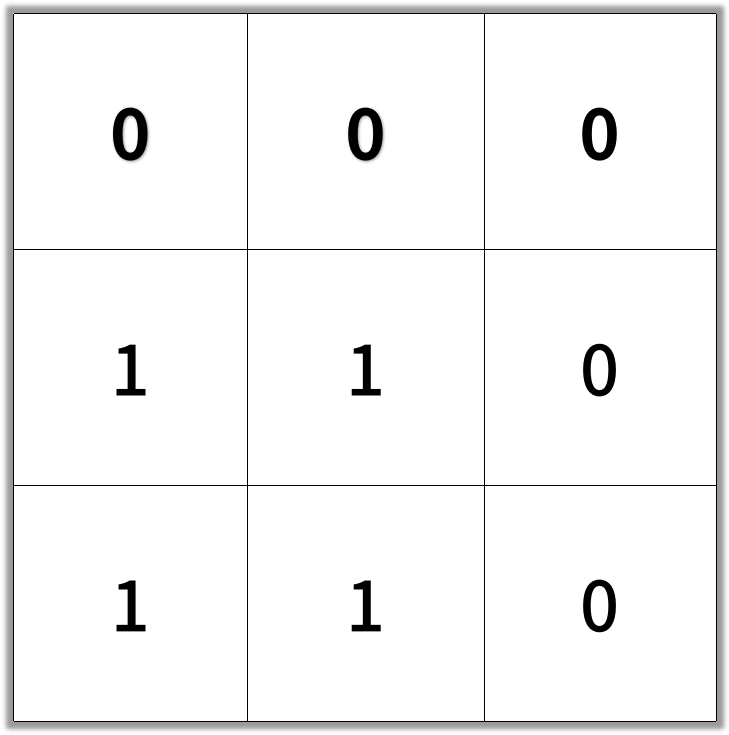## The challenge

In an N by N square grid, each cell is either empty (0) or blocked (1).

clear path from top-left to bottom-right has length `k` if and only if it is composed of cells `C_1, C_2, ..., C_k` such that:

• Adjacent cells `C_i` and `C_{i+1}` are connected 8-directionally (ie., they are different and share an edge or corner)
• `C_1` is at location `(0, 0)` (ie. has value `grid`)
• `C_k` is at location `(N-1, N-1)` (ie. has value `grid[N-1][N-1]`)
• If `C_i` is located at `(r, c)`, then `grid[r][c]` is empty (ie. `grid[r][c] ==&nbsp;0`).

Return the length of the shortest such clear path from top-left to bottom-right.  If such a path does not exist, return -1.

Example 1:

```Input: [[0,1],[1,0]]Output: 2```

Example 2:

```Input: [[0,0,0],[1,1,0],[1,1,0]]Output: 4```

Note:

1. `1 <= grid.length == grid.length <= 100`
2. `grid[r][c]` is `` or `1`

## The solution

 `````` 1 2 3 4 5 6 7 8 9 10 11 12 13 14 15 16 17 18 19 20 21 22 23 24 25 26 27 28 29 30 31 32 33 34 35 36 37 38 39 40 41 42 43 44 45 46 47 48 49 50 51 52 53 54 55 56 57 58 59 60 61 `````` ``````def shortestPathBinaryMatrix(self, grid: List[List[int]]) -> int: """ :type grid: List[List[int]] :rtype: int """ if grid != 0: return -1 q = [[0, 0, 1]] grid = 1 while len(q) != 0: # print(q) k, m, d = q.pop(0) # grid[k][m] = 1 if k == m == len(grid) - 1: return d # UP if k - 1 >= 0 and grid[k - 1][m] == 0: q.append([k - 1, m, d + 1]) grid[k-1][m] = 1 # DOWN if k + 1 < len(grid) and grid[k + 1][m] == 0: q.append([k + 1, m, d + 1]) grid[k+1][m] = 1 # LEFT if m - 1 >= 0 and grid[k][m - 1] == 0: q.append([k, m - 1, d + 1]) grid[k][m-1] = 1 # RIGHT if m + 1 < len(grid) and grid[k][m + 1] == 0: q.append([k, m + 1, d + 1]) grid[k][m+1] = 1 # TOP LEFT if k - 1 >= 0 and m - 1 >= 0 and grid[k - 1][m - 1] == 0: q.append([k - 1, m - 1, d + 1]) grid[k-1][m-1] = 1 # TOP RIGHT if k - 1 >= 0 and m + 1 < len(grid) and grid[k - 1][m + 1] == 0: q.append([k - 1, m + 1, d + 1]) grid[k-1][m+1] = 1 # BOTTOM LEFT if k + 1 < len(grid) and m - 1 >= 0 and grid[k + 1][m - 1] == 0: q.append([k + 1, m - 1, d + 1]) grid[k+1][m-1] = 1 # BOTTOM RIGHT if k + 1 < len(grid) and m + 1 < len(grid) and grid[k + 1][m + 1] == 0: q.append([k + 1, m + 1, d + 1]) grid[k+1][m+1] = 1 return -1 ``````